What are Calculated Volumes

In Delivery System Pro there are custom formulas that you can create on the flow relation lines for a particular measurement point in a delivery system for volumes. These volumes were calculated and used during the running of an allocation.

For PAE we created Calculated Volumes to replace this functionality. The difference between the two is that the Calculated Volumes will happen BEFORE an allocation is run and a record will be created in the table you select. The PAE engine will pick these volumes up just like they were entered by a user and be able to be in reports and queries.

For example, you may have a gas meter that you want to create a volume for by taking a volume from one meter and subtracting two other meter volumes from it and post in Daily Gas Estimates (DGE). You would set up the formula, select DGE as the target to post the data to and then place in a delivery system like normal.

Calculated Volumes in Action

Let’s take a look at Calculated volumes in action. Here we are looking at a delivery system where the highlighted meter (UC2021 W3 CHK) does not have a volume entered on it. We will create a calculated volume on this meter like the example mentioned above. The 3 meters that have circles around them (UC2021 W1 CHK, UC2021 W2 CHK, UC2021 INLET), we want to subtract UC2021 W1 CHK, and UC2021 W2 CHK DGE volumes from the DGE volume on meter UC2021 INLET and post on meter UC2021 W3 CHK in DGE as CKFLO.

So formula would look like this:

UC2021 W3 CHK = UC2021 INLET - (UC2021 W1 CHK + UC2021 W2 CHK)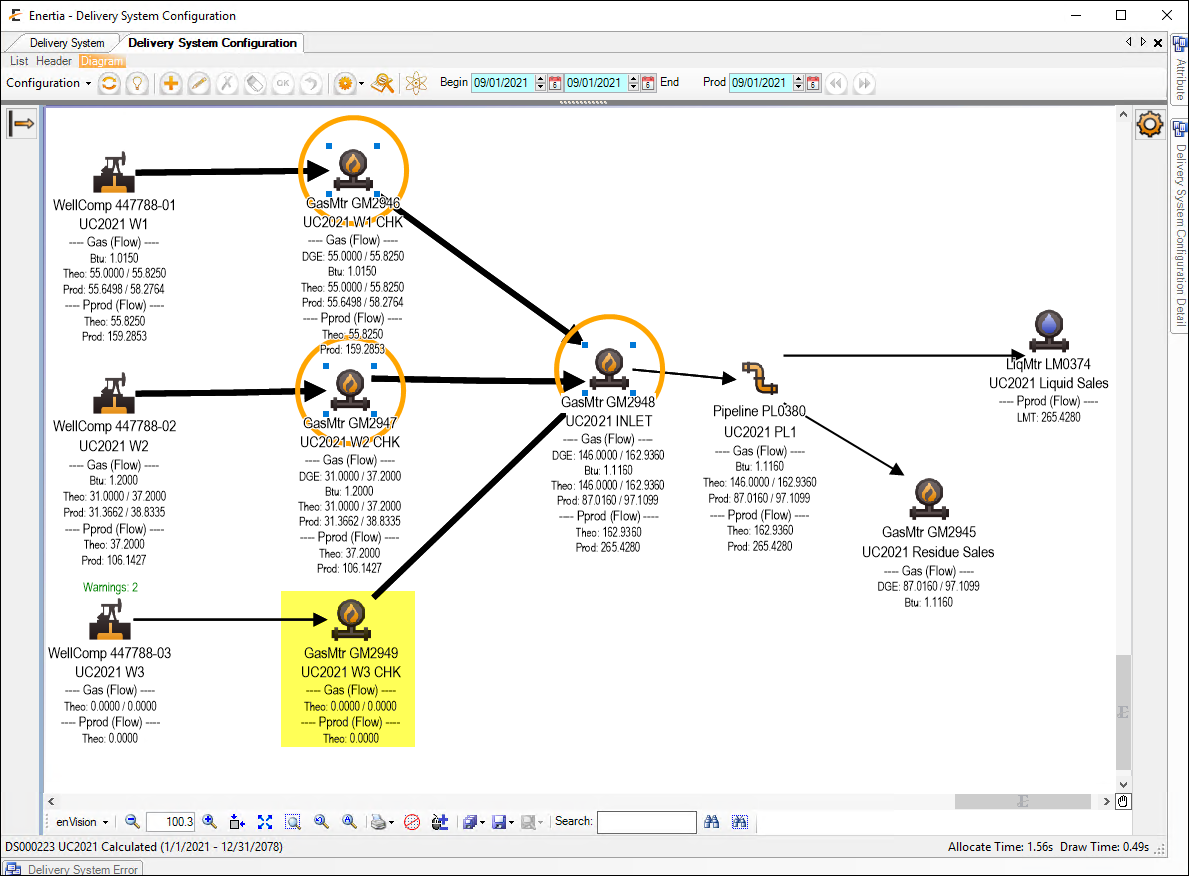To do this we will select the meter UC2021 W3 CHK and go to the Attributes tab and open Calculated Volumes.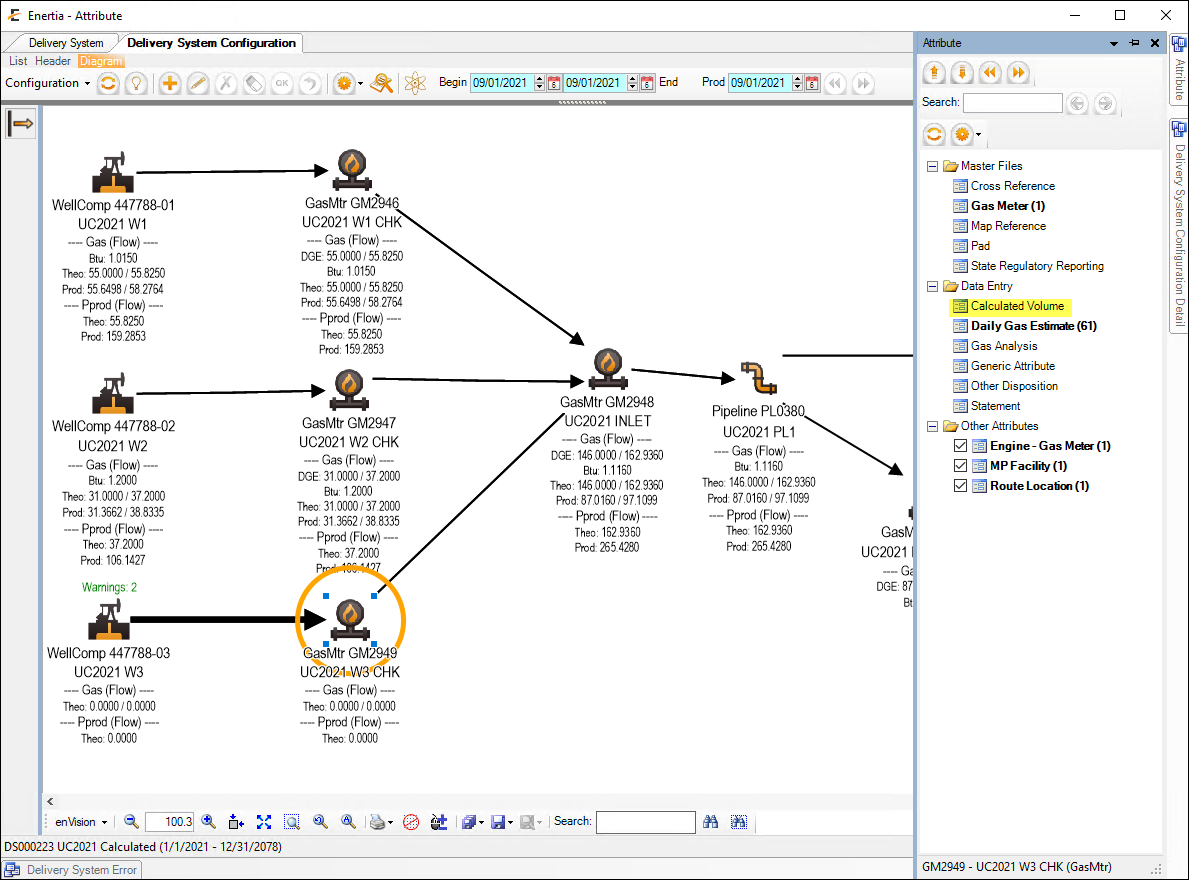The form opens in add mode. Enter in a name for the formula and then select the target Attribute for the result of the calculations. In this case the name is UC2021 DGE and we are putting results in DGE table.Then change Begin and End dates, Product UOM, Disposition and Pressure Base to below.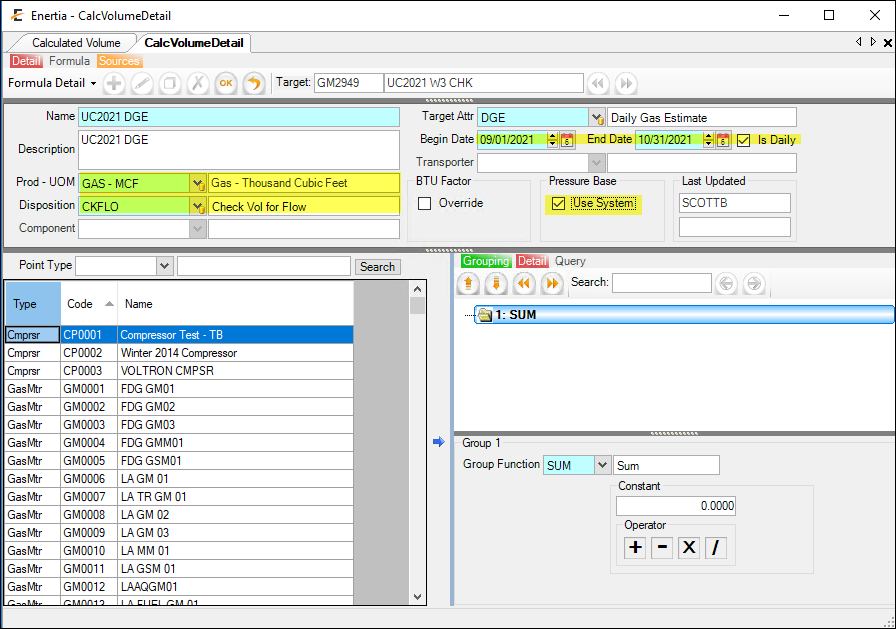At this point we have a date effective detail of what we want to create. Now we need to build the formula.

So from above we are wanting to create this formula: UC2021 W3 CHK = UC2021 INLET - (UC2021 W1 CHK + UC2021 W2 CHK)

What we see below in the Sources panel are all the production entities that are available to be selected and used in the formula. You can filter to a particular Type by using the point type drop-down or just type in the Search box.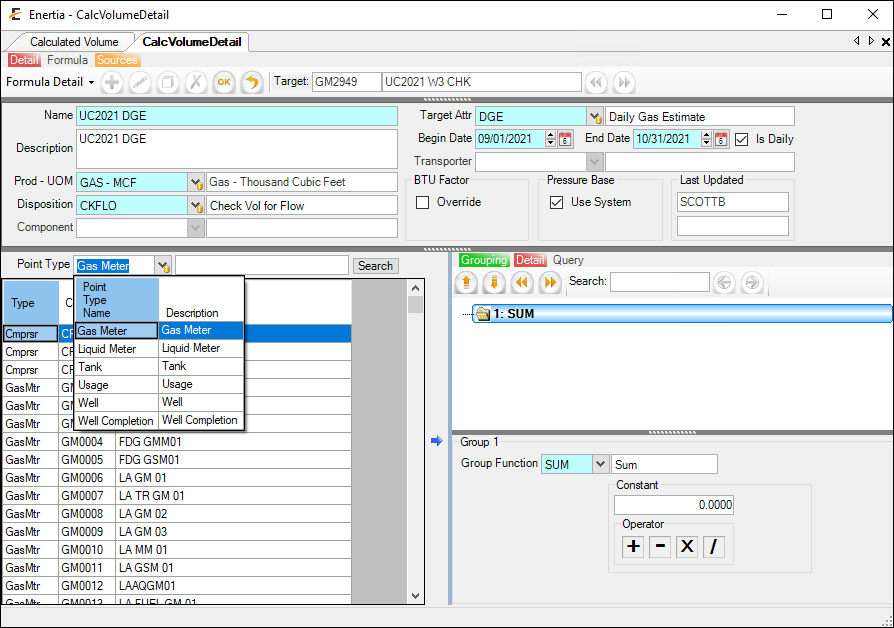We will just use search and type in UC2021 which will filter to below.On the right is the Grouping and detail. We will be using the SUM Grouping function in the Detail panel. This is controlled by the group Function drop-down and this will be group number 1. This what all entities in the group will have happen. Sum all volumes in the group.So the first meter we want to add is the UC2021 INLET meter. Select the meter and double click to move over or use the Right arrow.We now want to select which attribute to pull this data from so we will select the DGE table from the Attribute drop-down.It will default the Volume for you from the Column drop-down but if you press the drop-down it will list other options from this table. We will stay with the default numeric column from this table for selection which is the MCF. MMBTU will automatically be used as well in the calculation. We will then select GAS and CKFLO for the product and dispositions.So at this point we are going to SUM this meter even though there is only one and grab the volume from DGE.

Now we want to add another SUM group by right clicking in the grouping Panel and selecting Add Group. This will be the Group 2 which we will add the other two meters.We will then select a grouping Function of Subtract for the Operator (with Prev Group).Now we will add the other two meters just like the INLET meter for Group 2 and select DGE then save.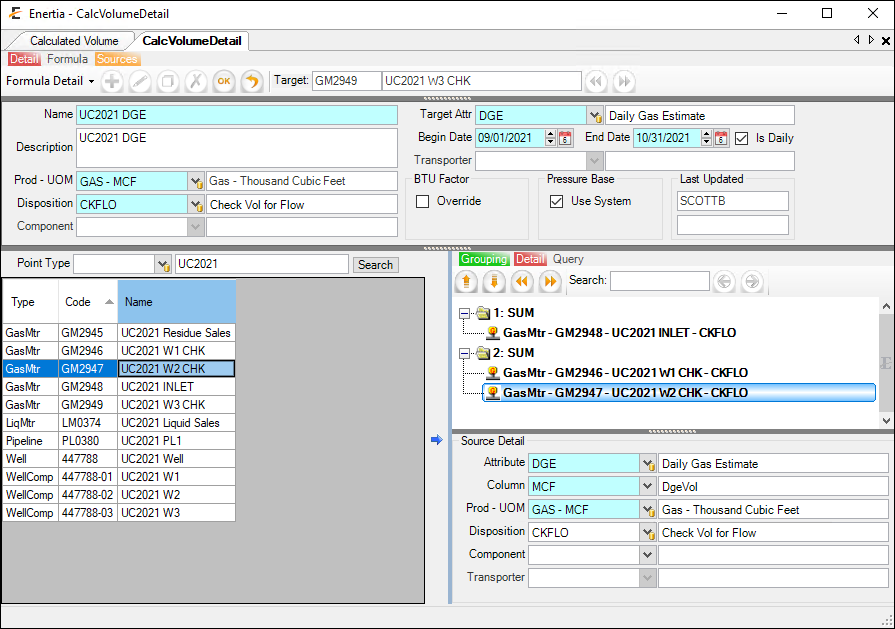While building the formula you can validate your results by turning on the Formula panel. This allows you to select dates and see what the value of the formula is so you can validate it is going to calculate correctly. So we changed the date for just 9/1/2021 and the results are correct for 60.We will now allocate 9/1/2021 in Designer and see if it creates the correct record. After we run we can see the volume of 60 has been created.This is what got created in DGE with a remark on which formula for this meter was used.